Jing, Y., Wang, X., Wang, D., and Yang, Q. (2020). "Optimization and modelling of Cd(II) removal from aqueous solution with composite adsorbent prepared from Alternanthera philoxeroides biochar and bentonite by response surface methodology," BioRes. 15(4), 9413-9428.

#### Abstract

Optimization and Modelling of Cd(II) Removal from Aqueous Solution with Composite Adsorbent Prepared from Alternanthera philoxeroides Biochar and Bentonite by Response Surface Methodology

Yande Jing,a,b Xuan Wang,a,b Dan Wang,a,b and Qianqian Yang a,b

Keywords: Biochar; Composite adsorbent; Response surface methodology; Cd(Ⅱ); Bentonite

Contact information: a: College of Geography and Tourism, Qufu Normal University, 80 Yantai Road, Rizhao, Shandong, 276826, China; b: Rizhao Key Laboratory of Territory Spatial Planning and Ecological Construction, 80 Yantai Road, Rizhao, Shandong, 276826, China;

* Corresponding author: jingyande@163.com

INTRODUCTION

Along with the rapid development of global industrialization and urbanization, a large amount of wastewater containing heavy metals is discharged into the water environment, especially in developing countries, which makes the pollution of heavy metals in water increasingly serious (Huang et al. 2016). Cadmium (Cd(Ⅱ)), mainly from the emissions of mining and chemical enterprises, is a common heavy metal in wastewater, which can cause severe toxicity even at low concentrations (Patar et al. 2016). Moreover, the difficult biodegradability and easy accumulability of Cd(Ⅱ) have caused a great threat to environmental safety and human health (Xu et al. 2016). It is imperative to search for an effective method to remove the Cd(Ⅱ) from industrial wastewater. The chemical methods are commonly used methods for Cd(Ⅱ) treatment, including neutralization, sulfide precipitation, adsorption, oxidation, ion exchange, electrolysis, etc (Purkayastha et al. 2014). The adsorption method has been widely used in the treatment of heavy metals in industrial wastewater due to its environmental friendliness, easy availability, and high adsorption capacity (Ma et al. 2016a; Zhang et al. 2019; Jing et al. 2020b).

Biochar has been found to have a well-developed pore structure and rich surface functional groups, which could remove heavy metals effectively in aqueous solution (Qian and Chen 2014; Son et al. 2018; Cao et al. 2019). Alternanthera philoxeroides (AP), an invasive and malignant weed in China, is an ideal raw material for biochar production (Wang et al. 2019; Yang et al. 2019). Bentonite (BE), a layered silicate mineral, has been applied to the adsorption of heavy metals gradually because of its large specific surface area and ion exchange capacity (Arbaoui and Boucherit 2014; Ren et al. 2014). At present, there are many studies on the application of the AP and BE as adsorbents alone in the remediation of heavy metal pollution (Huang et al. 2016; Ma et al. 2016b), but there are few studies on the combination of them into heavy metal composite adsorbents.

Traditional adsorption methods usually explore the influence of single variables such as pH value, temperature, and reaction time on adsorption efficiency (Hao et al. 2017). In order to determine the optimal adsorption level, the above methods are time consuming, high cost, and require a lot of manpower (Asachi and Marandi 2015). The response surface method can be used to analyze many variables that affect the adsorption effect of the experiment and establish a multiple quadratic regression equation model. Through fitting and optimizing the function relationship between multi-factor parameters and response value, the best adsorption process parameters were obtained (Biswas et al. 2019). It is one of the effective methods to optimize the best process parameters, reduce the number of experiments, and evaluate the level and interaction of various influencing factors method (Wang et al. 2017; Sharahi and Shahbazi 2017), which has been widely used in many areas of environmental pollution control and optimization experiments (Khattar and Shailza 2009; Nikraftar and Ghorbani 2017).

Composite adsorbents with different adsorption materials have shown different properties and heavy metal affinity. The authors’ previous studies have shown that composite adsorbent (BCB) preparation from the biochar (BC) and the BE had good results for the removal of Cd(Ⅱ) from aqueous solution (Jing et al. 2020a). However, the optimal adsorption parameters of the BCB for Cd(Ⅱ) removal have not been determined. In this study, the BCB was used as Cd(Ⅱ) adsorbent, and its physicochemical properties were characterized by Fourier-transform infrared spectroscopy (FTIR), X-ray diffraction (XRD), and scanning electron microscopy (SEM). Significant factors affecting the removal of Cd(Ⅱ) were screened out by the Plackett-Burman experiment (PBE), and the optimum conditions for Cd(Ⅱ) adsorption were determined by the RSM in aqueous solution.

EXPERIMENTAL

Materials

The AP was collected from a pond in Rizhao City, Shandong Province, China (35° 30’ N, 119°16’ E), and stored at room temperature after being air-dried, milled, and passed through a 2-mm sieve. The BE used in the experiment was purified from calcium-based BE. Its major chemical compositions were: SiO2 67.26% (quality fraction, the same below), Al2O3 16.30%, Fe2O3 3.16%, K2O 2.61%, CaO 4.54%, and MgO 1.57%. After simple physical purification, the calcium-based BE was screened using a 0.15-mm sieve. The Cd(Ⅱ) in aqueous solution was prepared from Cd(NO3)2∙4H2O, in which a small amount of NaNO3 was added as background electrolyte. Moreover, NaOH, HCl, and HNO3 used in the experiment were all analytically pure (Tianjin Hengxing Chemical Reagent Co., Ltd, Tianjin, China).

The surface morphologies of the adsorbents were determined using SEM (JSM-5600LV; JEOL Ltd., Tokyo, Japan). Fourier-transform infrared spectroscopy (Tensor 27; Bruker, Karlsruhe, Germany) was used to analyze the surface functional groups of the adsorbents. The scanning range was 4000 cm-1 to 500 cm-1, and the resolution was 4 cm-1. The mineral components in the adsorbents were analyzed by X-ray diffraction (XRD) (D8 advance; Bruker, Karlsruhe, Germany). The tube voltage was 20 to 60 kV and the tube current was 10 to 300 mA.

The pH values of the adsorbents were determined as follows: First, 2.5 g of the adsorbent was weighed and added into 50 mL of deionized water. The adsorbent was heated in airtight conditions, boiled for 5 min, and filtered, and 5 mL of primary filtrate was discarded. The pH of the residual liquid after cooling was measured using a pH meter.

Biochar Preparation

The biochar (BC) was prepared by temperature-controlled and oxygen-limited pyrolysis conditions at 350 ℃ for 2 h with a heating rate of 15 ℃∙min-1. The obtained biochar samples were ground and screened using a 100 mesh screen and labeled as BC.

The Plackett-Burman Experiment (PBE) Design

The PBE can screen out the factors that have significant influence on the adsorption effect from multiple factors. In this study, the PBE design of Cd(Ⅱ) adsorption was formulated for six factors using Minitab version 18 software (Minitab LLC, State College, PA, USA), and the experimental number selected was N = 12. Variables X1, X2, X3, X4, X5, and X6 represented initial solution pH, initial concentration of Cd(Ⅱ), dosage of composite adsorbent, adsorption temperature, adsorption time, and shaking frequency, respectively, as a single factor affecting the adsorption capacity. Two levels of low value (-1) and high value (+1) were determined for each factor, as shown in Table 1. The response value was the adsorption rate of the adsorbent for Cd(Ⅱ). Concentrations of Cd(Ⅱ) in the filtrate after dilution were determined by an atomic absorption spectrophotometer (GFA-7000A; Shimadzu, Kyoto, Japan) and the response value was calculated by Eq. 1,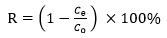(1)

where represents the response value, i.e., the adsorption rate of Cd(Ⅱ) (%), Cis the concentration of Cd(Ⅱ) in the solution after adsorption (mg∙L-1), and C0 is the concentration of Cd(Ⅱ) in the initial solution (mg∙L-1).

Table 1. Factors and Levels of the PBE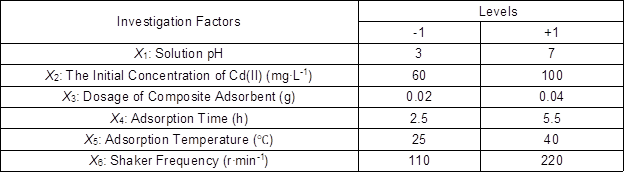The adsorption capacity of BCB, BC, and BE was calculated using Eq. 2, and the data of adsorption were fitted to both the Langmuir model (Eq. 3) and Freundlich model (Eq. 4),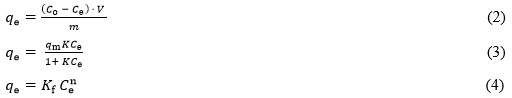where qe is the equilibrium adsorption capacity (mg∙g-1), Cand Ce are the initial and equilibrium concentration (mg∙g-1), respectively. Variable V is the volume of solution (L), m is the weight of adsorbents (g), qm is the maximum adsorption (mg∙g-1), is the adsorption equilibrium constant (L∙mg-1), Kis the adsorption capacity (mg∙g-1), and n is the Freundlich constant.

Response Surface Optimization of Adsorption Experiment Design

The optimum conditions for Cd(Ⅱ) adsorption in aqueous solutions were optimized by the multi-factor and multi-level Box-Behnken response surface optimization method. Contour maps and three-dimensional stereograms were established to test the adaptability and significance of the model, thereby optimizing the adsorption parameters of composite adsorbent for Cd(Ⅱ). The second-order model formula of the RSM was derived from the Numerical Solution function of Design-Expert 8.0 software (Stat-Ease, Inc., Minneapolis, MN, USA) as follows,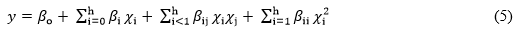where y is the predicted response variable (%), β0βi, and βij are the constant regression coefﬁcients of the model, and χi and χj are the independent variables in the form of coded values.

RESULTS AND DISCUSSION

Physicochemical Properties of Absorbents

The FTIR spectra of the BCB, BC, and BE are shown in Fig. 1. The recognition of the obtained spectra had a typical peak of -OH group at 3615 to 3423 cm-1 (Yu et al. 2018). The absorption peak near the 2990 to 2835 cm-1 was an aliphatic -CH2 stretching vibration peak. However, there was no similar absorption peak in the BE spectrum, indicating no aliphatic group in the BE. The peak at 1625 cm-1 represented the stretching vibrations of C=O and C=C, corresponding to aromatic characteristics. Compared with BE, BCB, and BC had more obvious vibration peaks, indicated that the BCB and BC had more carbonyl functional groups and complex aromatic structures. The stretching vibration peak near 1395 to 1303 cm-1 represented the C-O. The sharp vibrational peak at 1039 cm-1 in the BCB and BE was caused by structural vibration of Si-O tetrahedron and Al-O octahedron of the BE (Liu et al. 2011), which was an important feature of the BE particle loaded on the surface of biochar. The stretching vibration peak near band 753 cm-1 was considered to the vibration of inorganic functional group Si-O-Si (Qian and Chen 2013). In this study, the number of functional groups of the BCB, BC, and BE might significantly affect the adsorption capacity of Cd(Ⅱ).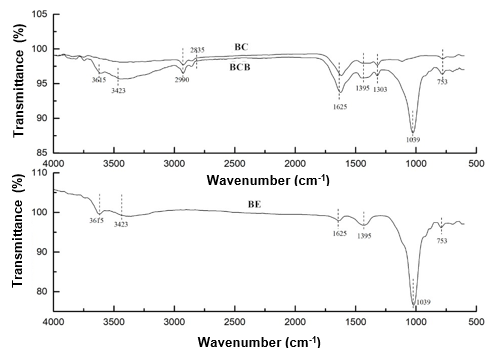Fig. 1. FTIR spectra of BC, BCB, and BE

SEM images of BCB, BC, and BE at different magnification (500 times (a, d, g), 1000 times (b, e, h), and 2000 times (c, f, i), from left to right) are shown in Fig. 2.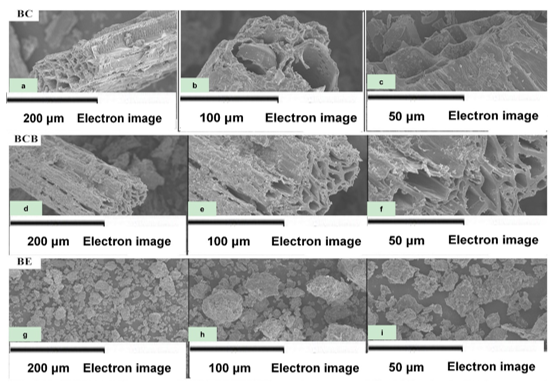Fig. 2. SEM images of the BC (a, b, c), BCB (d, e, f), and BE (g, h, i) before and after Cd(II) adsorption by the BCB

From the micrographs, the samples of BC and BCB had typical microscopic morphology of plant-derived biochar: intact tubular structure and distinct pore structure (Keiluweit et al. 2010; Lian and Xing 2017). These better microporous and developed pore structures (with more holes and larger aperture) facilitated the binding of Cd(Ⅱ) with more adsorption sites. The BC had a thickly layered structure and smooth external morphology, while the surface of the BCB was covered with a layer of layered sediments similar to the morphology of the BE, indicating that BE particles were loaded on the surface of biochar successfully. Compared with the BC, there was no significant change in SEM images of the BCB, which was attributed to the fact that the BE particles were mainly loaded on biochar by physical methods during the preparation of BCB. The SEM images of the BE were different from the BC and BCB, and its morphologies were agglomerated structure with large voids. This might be the result of the active surface polarity and surface energy of the BE, which was conducive to the preparation of composite adsorbents (Bilgic et al. 2014) with high adsorption performance by modification or compounding with organic/inorganic materials.

The XRD spectrum of BCB before (a) and after (b) Cd(Ⅱ) adsorption is shown in Fig. 3. The relatively broad amorphous diffraction peaks did not appear in the range of 10° to 50°, most of which were sharp crystal diffraction peaks. For example, the 2θ = 26.5° might be the characteristic diffraction peak of graphite crystal. It could be inferred that higher polymeric aromatic rings might contain in the carbon molecular structure (Popova 2018). In the spectrum of BCB + Cd, the sharp diffraction peaks’ intensity between 2θ = 20° to 22° decreased, which might be caused by the separation of the BE particles loaded on the surface of biochar from biochar or the oxidation of oxygen-containing functional groups during the vibration process (Fan et al. 2010). Additionally, the new diffraction peaks (α) emerged, indicating that the new precipitated materials were formed after Cd(Ⅱ) adsorption and the primary mechanism of Cd(Ⅱ) adsorption by the BCB was surface precipitation (Zhou et al. 2013).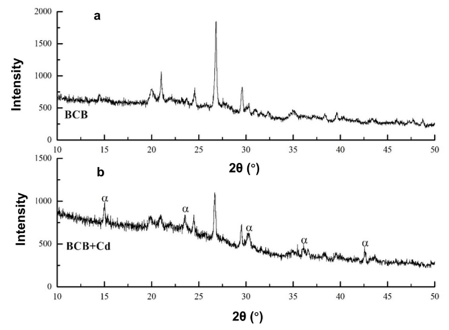Fig. 3. XRD spectrum before (a) and after (b) Cd(II) adsorption by the BCB

Plackett-Burman Experiment Analysis

The response values of the PBE design are shown in Table 2, and the variance analysis results of the regression model (ANOVA) showed that the P-value of the model was 0.0054 (P 0.05), indicating that the model was statistically significant within the selected level range (Deng et al. 2017). According to the P-values of the six factors, the factors affecting adsorption rate were X1 > X2 > X> X> X4 > X6 in order of influence. The significant factors (P < 0.05): X1, X2, and X3i.e., the pH, the initial concentration, and the adsorbent dosage, were assessed as crucial factors in the steepest climbing and response surface optimization analysis experiments. Factors X4, X5, and X6 (P > 0.05) had no significant difference. However, that did not mean that they did not affect the Cd(Ⅱ) adsorption in the solution. The data of the model coefficient effect showed that factor X1 and X3 were positively correlated with the response value and negatively correlated with the X2.

The determination coefficient R2 = 0.9861 indicated that the model had high significance, accuracy, and reliability. In addition, the adjusted determination coefficient R2adj = 0.9693 indicated that the model can explain 96.93% of variability of the experimental data.

Table 2. The Results of the PBE Design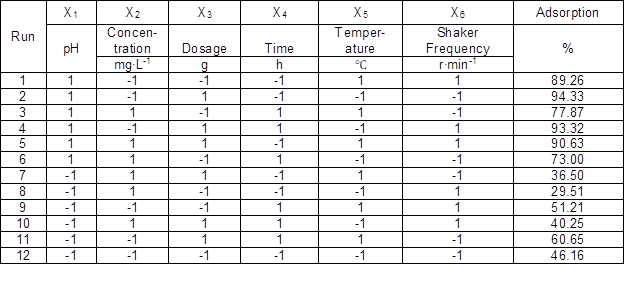Significant Single Factor Steepest Climbing Design

Based on the influence of each factor, the steepest climbing experiment design was adopted to approach the optimal value region efficiently. The significant factors were selected from the PBE analysis of variance, and the gradient size and climbing direction could be determined by the effective value of significant factors in the PBE. The design of the steepest climbing experiment was formulated according to the following steepest ascent gradients of a key single factor: the critical single factor pH (3, 4, 5, 6, and 7), the initial concentration of cadmium (20, 40, 70, 90, and 120 mg∙L-1), and the dosage of composite adsorbent (0.01, 0.02, 0.03, 0.04, and 0.05 g).

Effect of the pH, Initial Concentration, and Adsorbent Dosage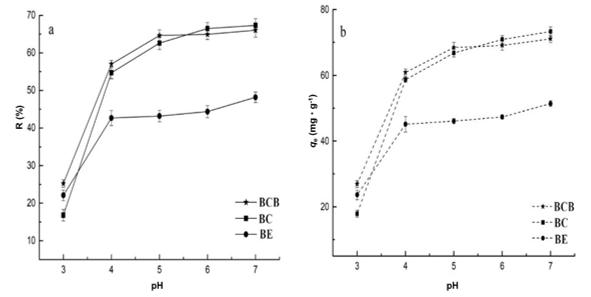Fig. 4. Effect of pH on Cd(II) adsorption under temperature 25 °C, initial concentration 80 mg∙L-1, contact time 24 h, dose 0.03 g: (a) the adsorption rate of Cd(II) and (b) the adsorption capacity of Cd(II)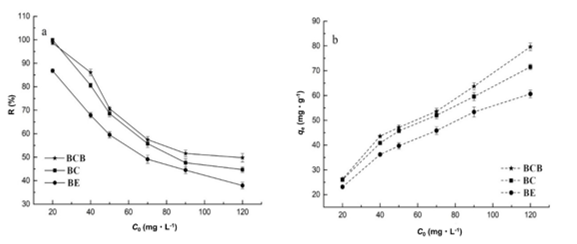Fig. 5. Effect of initial concentration on Cd(II) adsorption under temperature 25 °C, contact time 24 h, dose 0.03 g, and pH at 4: (a) the adsorption rate of Cd(II) and (b) the adsorption capacity of Cd(II)

Figure 5 indicates the changing trend of the R and qe of Cd(Ⅱ) adsorbed by the BCB, BC, and BE with the Cd(Ⅱ) initial concentration. The results showed that the qe increased with the increase of the Cd(Ⅱ) initial concentration, while the adsorption rate was the opposite. The adsorbent could provide sufficient adsorption sites at a lower Cd(Ⅱ) initial concentration. However, the active adsorption sites decreased gradually as the Cd(Ⅱ) initial concentration increased, and the adsorption process changed into pore adsorption, resulting in a decrease of adsorption rate (Liu et al. 2010).

The fitting parameters of Langmuir and Freundlich models are listed in Table 3. The better appropriate prediction for Cd(Ⅱ) adsorption was provided by the Freundlich model, as it had a higher coefficient of determination (R2) than the Langmuir model., and the adsorption capacity would rise continuously with the increase of the initial concentration of Cd(Ⅱ) (Wang et al. 2018). It was noted that R2 of the Langmuir model was also very high. Since Cd(Ⅱ) adsorption involved specific adsorption and non-specific adsorption, and was affected by physicochemical properties of the composite adsorbent, it was also very complex to describe the law from limited data by mathematical methods. Therefore, the hypothesis of the Freundlich model did not negate the hypothesis of Langmuir model.

Table 3. Langmuir and Freundlich Fitting Results on Cd(II) Adsorption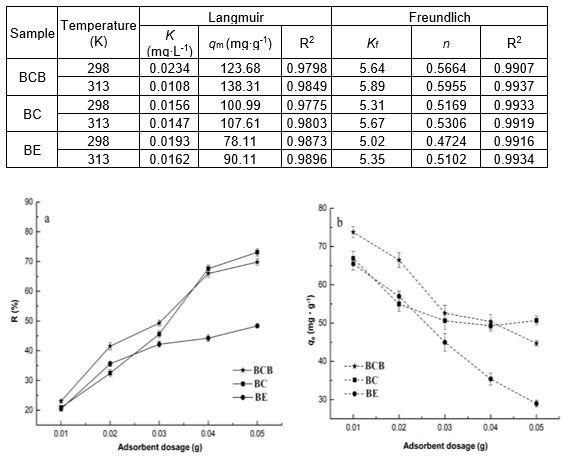Fig. 6. Effect of the adsorbent dosage on Cd(II) adsorption under temperature 25 °C, initial concentration 80 mg∙L-1, contact time 24 h, and pH at 4: (a) the adsorption rate of Cd(II) and (b) the adsorption capacity of Cd(II)

The influence of the adsorbent dosage on Cd(Ⅱ) adsorption was evaluated. It could be seen from Fig. 6 that the R values of BCB and BC increased from 20.0% to more than 70% when the absorbent dose was increased from 0.01 to 0.05 g. On the contrary, the qe decreased significantly, which might be that the active surface area of the adsorbent decreased with continued increment in the adsorbent dosage within a certain volume (Wang et al. 2010). More active adsorption sites and functional groups could be provided when the adsorbent dosage increased, which promoted the adsorption effect. Furthermore, the adsorption effect of BCB was the best when the dosage was less than 0.04 g, which might be related to the change in the adsorption properties of the BE.

Based on the results of the PBE and the steepest climbing experiment, it was determined that the pH, the initial concentration, and the adsorbent dosage were the main factors affecting the adsorption effect of Cd(Ⅱ). Therefore, the design of the Box-Behnken response surface optimization experiment was made based on the following codes and levels of independent variables: the pH (3 (code, -1), 5 (code, 0), 7 (code, +1)), the initial concentration of cadmium (60 mg∙L-1 (code, -1), 80 mg∙L-1 (code, 0), 100 mg∙L-1 (code, +1)), and the dosage of composite adsorbent (0.02 g (code, -1), 0.03 g (code, 0), and 0.04 g (code, +1)).

The Box-Behnken design matrix consisted of 17 trials, as shown in Table 4. The second-order polynomial equation (Ym) was established by response surface regression analysis based on Eq. 2:

Y75.46 + 14.74+ 8.67 3.96C – 0.14AC + 4.34AB + 2.69BC  12.36A 2.48B 1.22C2 (6)

Table 4. The Results of Box-Behnken Design Matrix in Coded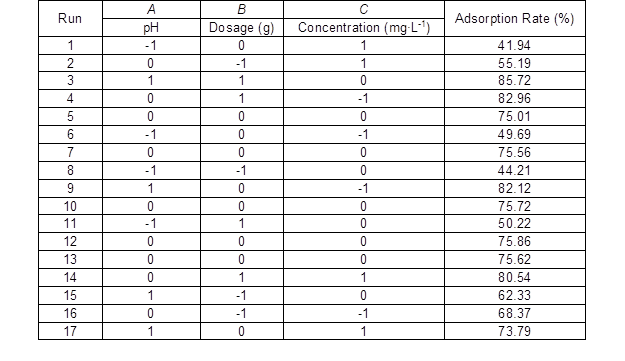The ANOVA results of response values showed that the regression model had a P < 0.0001 and the “lack of fit P-value” of 0.36, i.e., the model was very signiﬁcant. Number 65.21 of the given F-value and 0.99 of the R2 value revealed that the model was suitable for describing the relationship between each independent variable, i.e., pH (A), Cd(Ⅱ) initial concentration (B), dosage (C), and response value. In conclusion, the response surface model was suitable for optimizing the adsorption of CD(Ⅱ) adsorption on the composite adsorbents.

Because the P-values of all coefﬁcients were P < 0.05, expect AC and C2, it meant that these were signiﬁcant or very signiﬁcant, including linear effects (AB, and C), interactive effects (AB and BC) and quadratic terms (A2 and B2). Thus, the influences of three key factors on the response value were not only the linear effect but also the interactive and quadratic effect.

In order to intuitively express the influences of various factors on the response value, the response surface contours are shown in Figs. 7a to 7f.